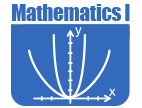# Math - Secondary Math I

IntroductionTHE FUNDAMENTAL PURPOSE OF SECONDARY MATHEMATICS I is to formalize and extend the mathematics that students learned in the middle grades. The critical areas, organized into units, deepen and extend understanding of linear relationships, in part by contrasting them with exponential phenomena, and in part by applying linear models to data that exhibit a linear trend. Secondary Mathematics I uses properties and theorems involving congruent figures to deepen and extend understanding of geometric knowledge from prior grades. The final unit in the course ties together the algebraic and geometric ideas studied. The Mathematical Practice Standards apply throughout each course and, together with the content standards, prescribe that students experience mathematics as a coherent, useful, and logical subject that makes use of their ability to make sense of problem situations.

CRITICAL AREA 1: By the end of eighth grade, students have had a variety of experiences working with expressions and creating equations. Students continue this work by using quantities to model and analyze situations, to interpret expressions, and by creating equations to describe situations.

CRITICAL AREA 2: In earlier grades, students define, evaluate, and compare functions, and use them to model relationships between quantities. Students will learn function notation and develop the concepts of domain and range. They move beyond viewing functions as processes that take inputs and yield outputs, and start viewing functions as objects in their own right. They explore many examples of functions, including sequences; they interpret functions given graphically, numerically, symbolically, and verbally, translate between representations, and understand the limitations of various representations. They work with functions given by graphs and tables, keeping in mind that, depending upon the context, these representations are likely to be approximate and incomplete. Their work includes functions that can be described or approximated by formulas, as well as those that cannot. When functions describe relationships between quantities arising from a context, students reason with the units in which those quantities are measured. Students build on and informally extend their understanding of integer exponents to consider exponential functions. They compare and contrast linear and exponential functions, distinguishing between additive and multiplicative change. They interpret arithmetic sequences as linear functions and geometric sequences as exponential functions.

CRITICAL AREA 3: By the end of eighth grade, students have learned to solve linear equations in one variable and have applied graphical and algebraic methods to analyze and solve systems of linear equations in two variables. This area builds on these earlier experiences by asking students to analyze and explain the process of solving an equation and to justify the process used in solving a system of equations. Students develop fluency writing, interpreting, and translating between various forms of linear equations and inequalities, and using them to solve problems. They master the solution of linear equations and apply related solution techniques and the laws of exponents to the creation and solution of simple exponential equations. Students explore systems of equations and inequalities, and they find and interpret their solutions. All of this work is grounded on understanding quantities and on relationships between them.

CRITICAL AREA 4: This area builds upon students’ prior experiences with data, providing students with more formal means of assessing how a model fits data. Students use regression techniques to describe approximately linear relationships between quantities. They use graphical representations and knowledge of the context to make judgments about the appropriateness of linear models. With linear models, they look at residuals to analyze the goodness of fit.

CRITICAL AREA 5: In previous grades, students were asked to draw triangles based on given measurements. They also have prior experience with rigid motions (translations, reflections, and rotations) and have used these to develop notions about what it means for two objects to be congruent. In this unit, students establish triangle congruence criteria, based on analyses of rigid motions and formal constructions. They solve problems about triangles, quadrilaterals, and other polygons. They apply reasoning to complete geometric constructions and explain why they work.

CRITICAL AREA 6: Building on their work with the Pythagorean Theorem in eighth grade to find distances, students use a rectangular coordinate system to verify geometric relationships, including properties of special triangles and quadrilaterals and slopes of parallel and perpendicular lines.The Online Core Resource pages are a collaborative project between the Utah State Board of Education and the Utah Education Network. If you would like to recommend a high quality resource, contact Trish French (Elementary) or Lindsey Henderson (Secondary). If you find inaccuracies or broken links contact resources@uen.org.